Algebra 2 5-1 Complete Lesson: Polynomial Functions
starstarstarstarstarstarstarstarstarstar
3.5 (2 ratings)
by Matthew Richardson
| 29 Questions
Note from the author:
A complete formative lesson with embedded slideshow, mini lecture screencasts, checks for understanding, practice items, mixed review, and reflection. I create these assignments to supplement each lesson of Pearson's Common Core Edition Algebra 1, Algebra 2, and Geometry courses. See also mathquest.net and twitter.com/mathquestEDU.The outlined content above was added from outside of Formative.
1
10 pts
Solve It! The first column shows a sequence of numbers.
◆ For 1st differences, subtract consecutive numbers in the sequence: -6-(-4) = -2, 4-(-6) = 10, and so on.
◆ For 2nd differecnes, subtract consecutive first differences.
◆ For 3rd differences, subtract consecutive second differences.

If the pattern continues, what is the 8th number in the first column?

8
502
1074
8482
2
10 pts
Problem 1 Got It?
A
B
C
D3
3
10 pts
Problem 1 Got It?
A
B
C
D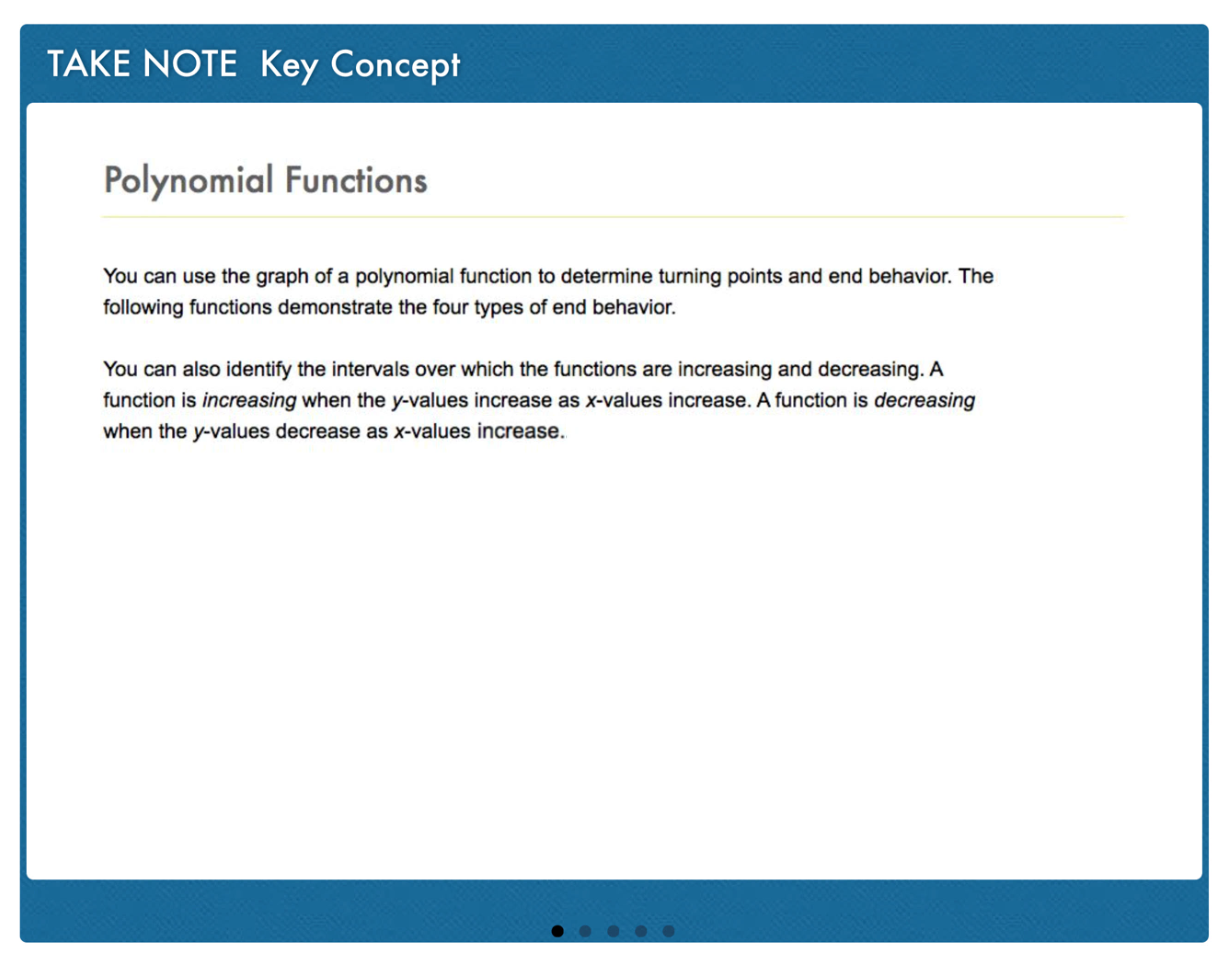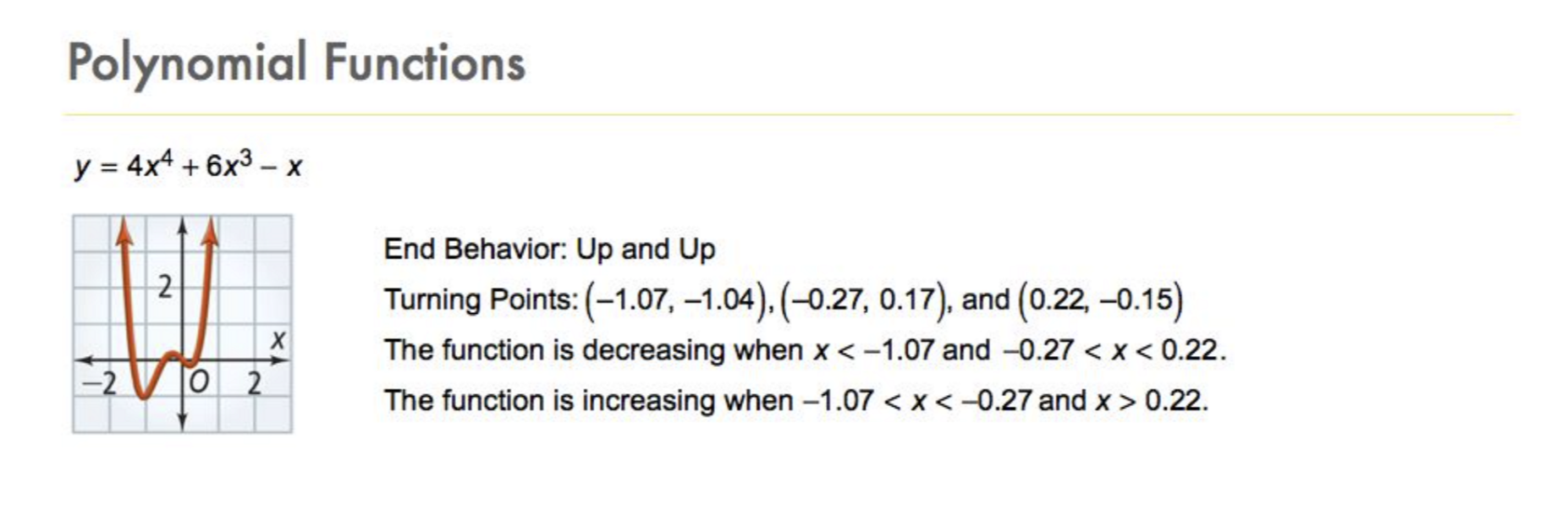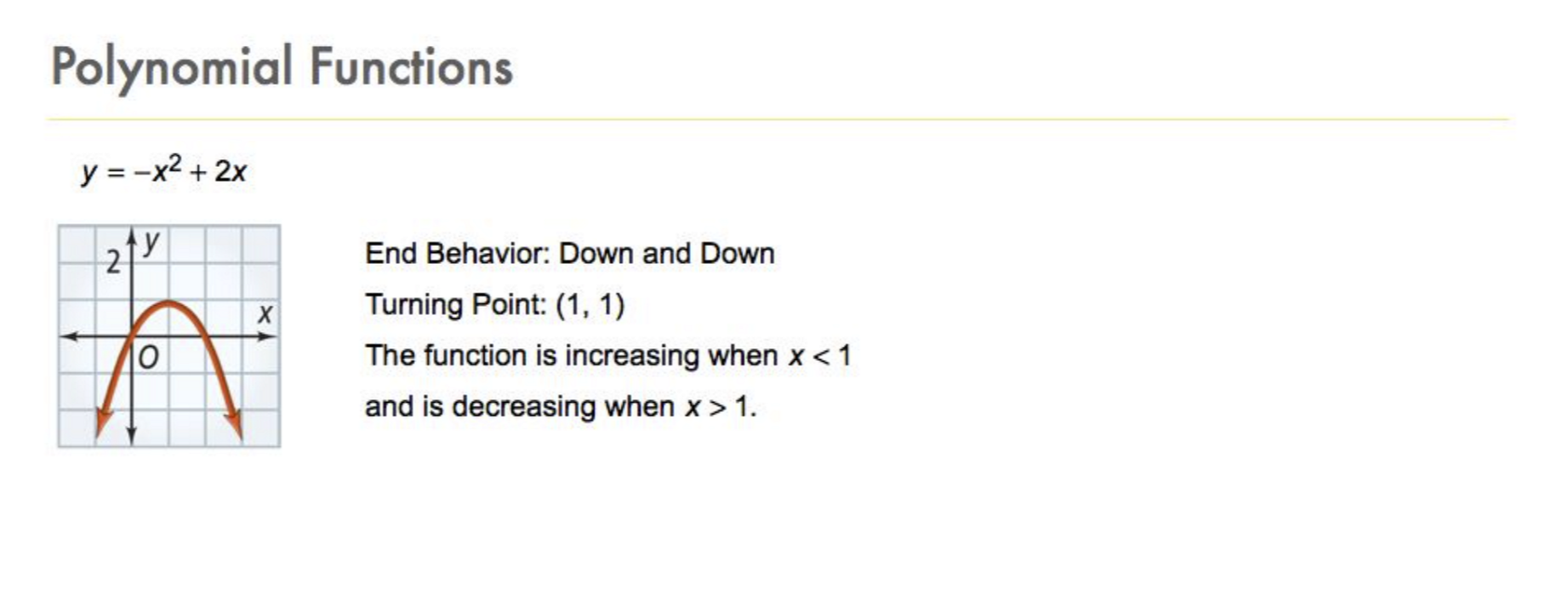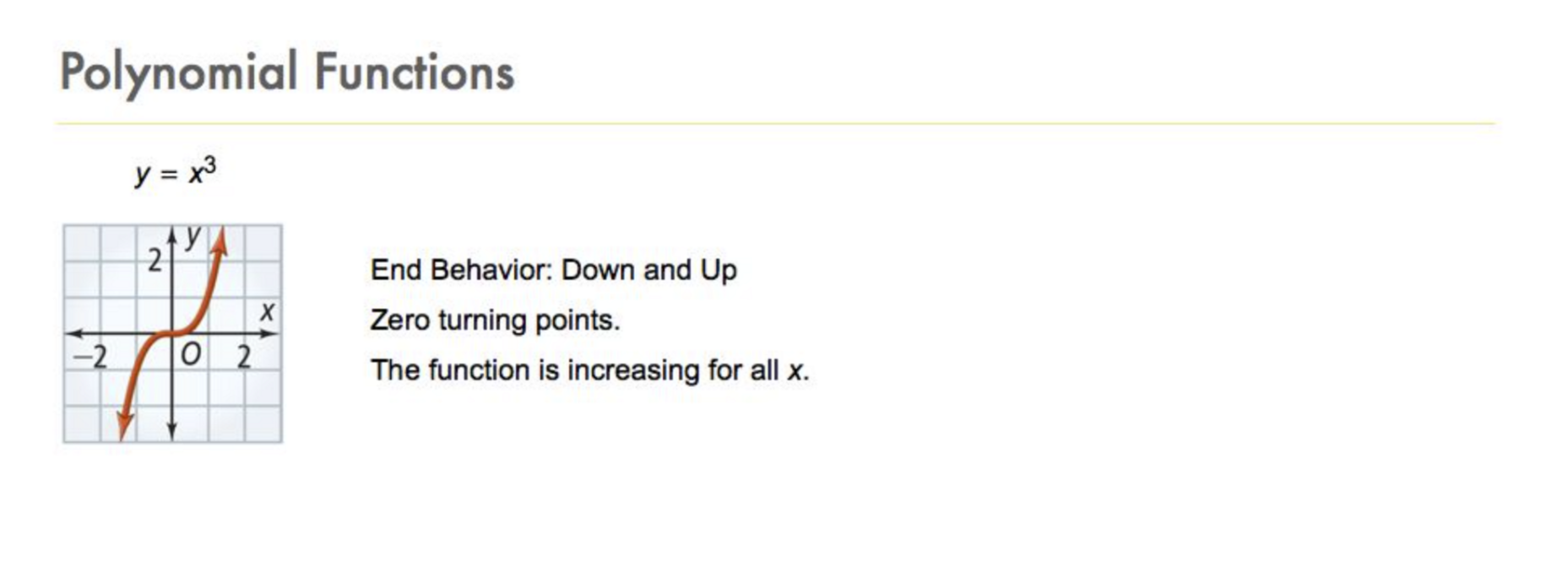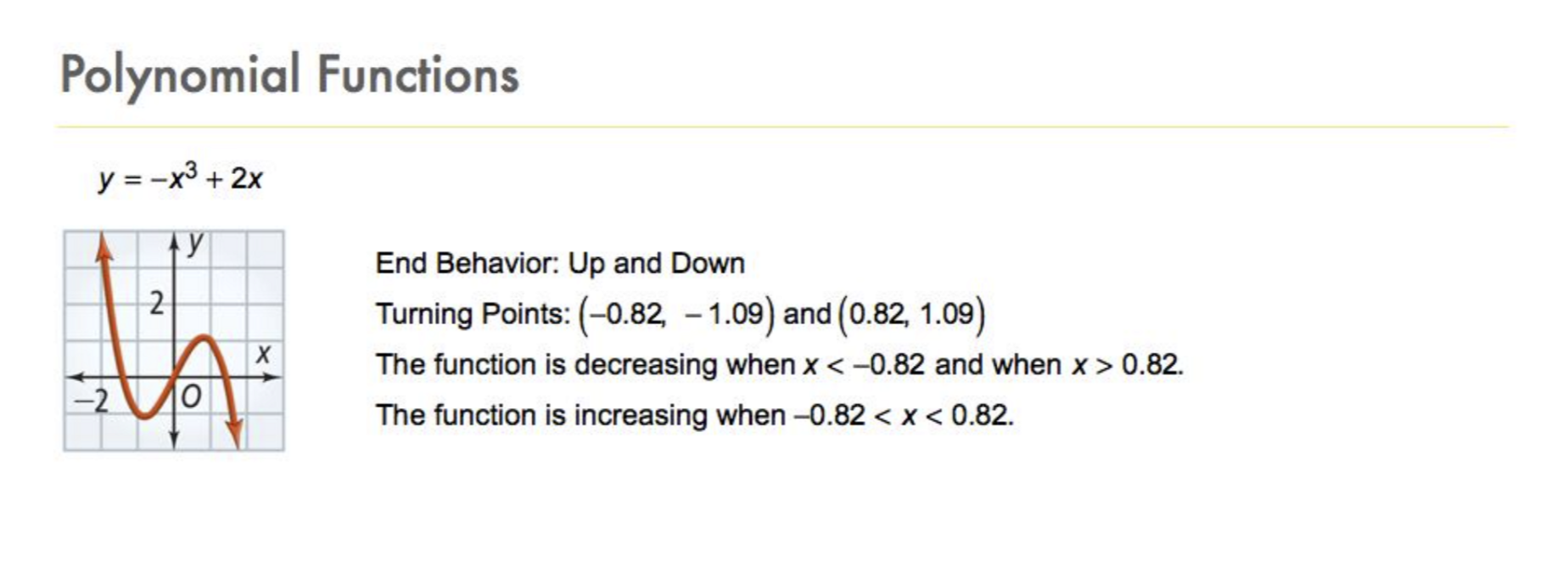4
4
10 pts
Problem 2 Got It?
A
B
C
D
5
10 pts
Problem 3 Got It? Identify the end behavior and turning points of the graph of the function as well as any intervals in which the graph is increasing or decreasing.
• up and down
• down and up

• End behavior
• Turning points
• Increasing
• Decreasing
6
10 pts
Problem 3 Got It? Graph the function. Zoom and pan your graph to establish an appropriate viewing window. Note the end behavior and turning points of the graph and any intervals in which the graph is increasing or decreasing. You may revise your responses to the previous item, as needed.
7
10 pts
Problem 3 Got It? Identify the end behavior and turning points of the graph of the function as well as any intervals in which the graph is increasing and/or decreasing.
• up and down
• down and up

• no turning points

• End behavior
• Turning points
• Increasing
8
10 pts
Problem 3 Got It? Graph the function. Zoom and pan your graph to establish an appropriate viewing window. Note the end behavior and turning points of the graph and any intervals in which the graph is increasing or decreasing. You may revise your responses to the previous item, as needed.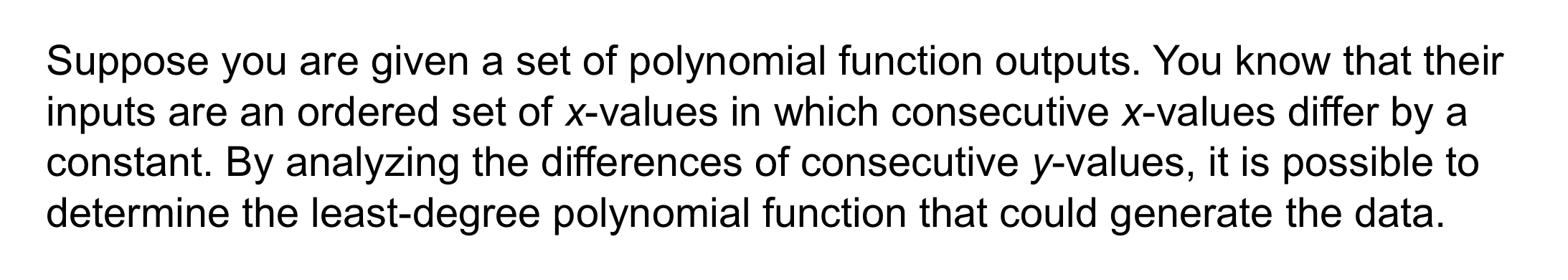9
10
9
10 pts
Problem 4 Got It? What is the degree of the polynomial function that generates the data in the table?
2
3
4
5
10
10 pts
Problem 4 Got It? Reasoning: What is an example of a polynomial function whose fifth differences are constant, but whose fourth differences are not constant?11
11
10 pts
A
B
C
D12
12
10 pts
A
B
C
D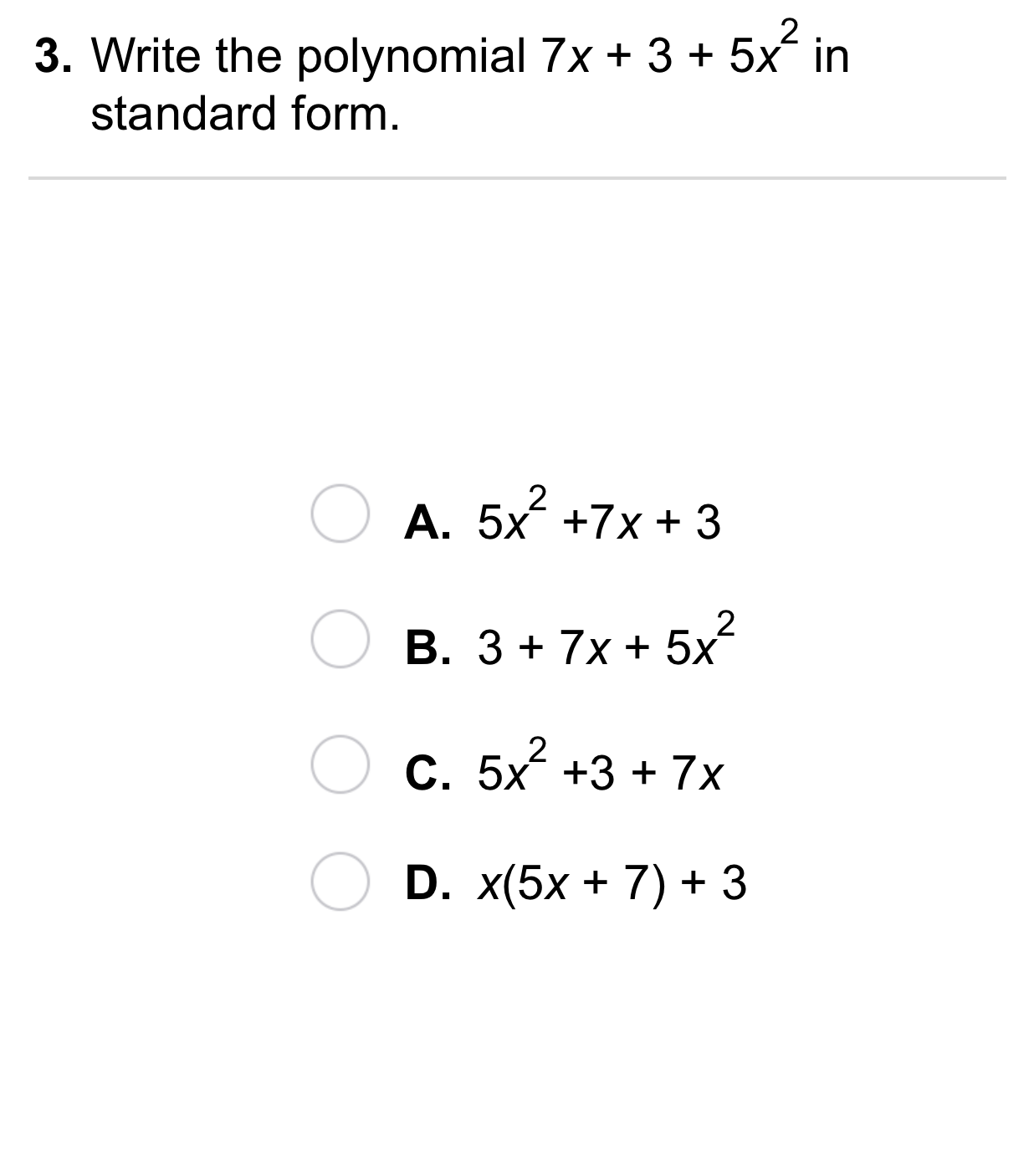13
13
10 pts
A
B
C
D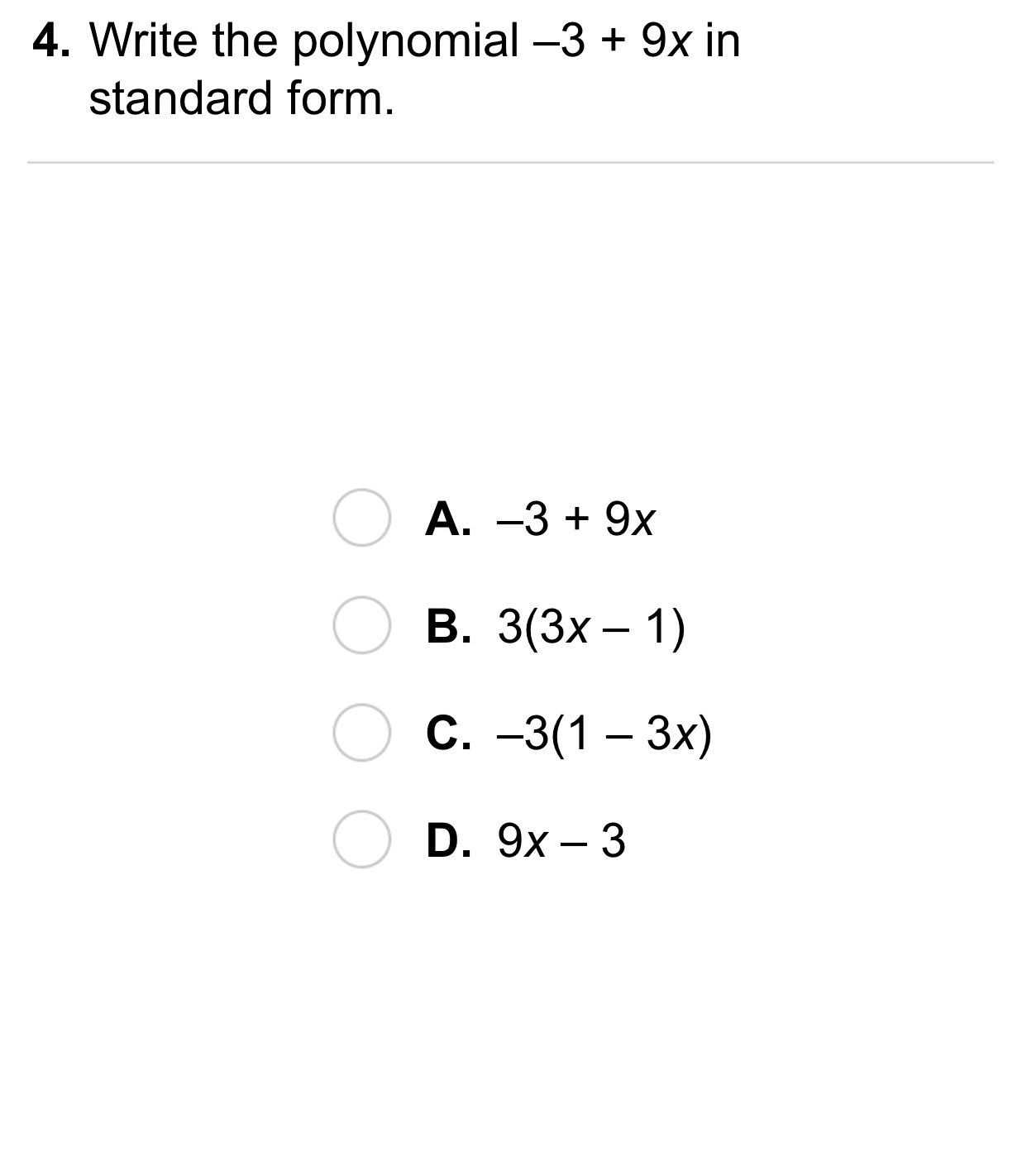14
14
10 pts
A
B
C
D15
10 pts
Vocabulary: Describe the end behavior of the graph of the function.
up and down
down and down
down and up
up and up
16
10 pts
Reasoning: Can the graph of a polynomial function be a straight line? If so, give an example.18
10 pts
Review Lesson 2-4: Match each equation in the left column with its standard form version in the right column.

19
10 pts
Review Lesson 4-4: Factor the quadratic expression.
(x + 6)(x + 1)
(x + 3)(x + 4)
(x + 5)(x + 2)
(x - 7)(x - 1)
20
10 pts
Review Lesson 4-4: Factor the quadratic expression.
21
10 pts
Review Lesson 4-4: Factor the quadratic expression.22
10 pts
Vocabulary Review: Tag each quadratic espression based on whether or not it is in standard form.
• standard form
• NOT standard form23
10 pts
Use Your Vocabulary: Identify the polynomial expression.

• Polynomial expression
24
10 pts
Vocabulary Review: Identify the graphs that can be represented by a polynomial.

• Can be represented by a polynomial
25
5 pts
Use Your Vocabulary: How many terms are in the polynomial ?
Enter only a number.
26
5 pts
Use Your Vocabulary: How many terms are in the polynomial ?
Enter only a number.
27
5 pts
Use Your Vocabulary: How many terms are in the polynomial ?
Enter only a number.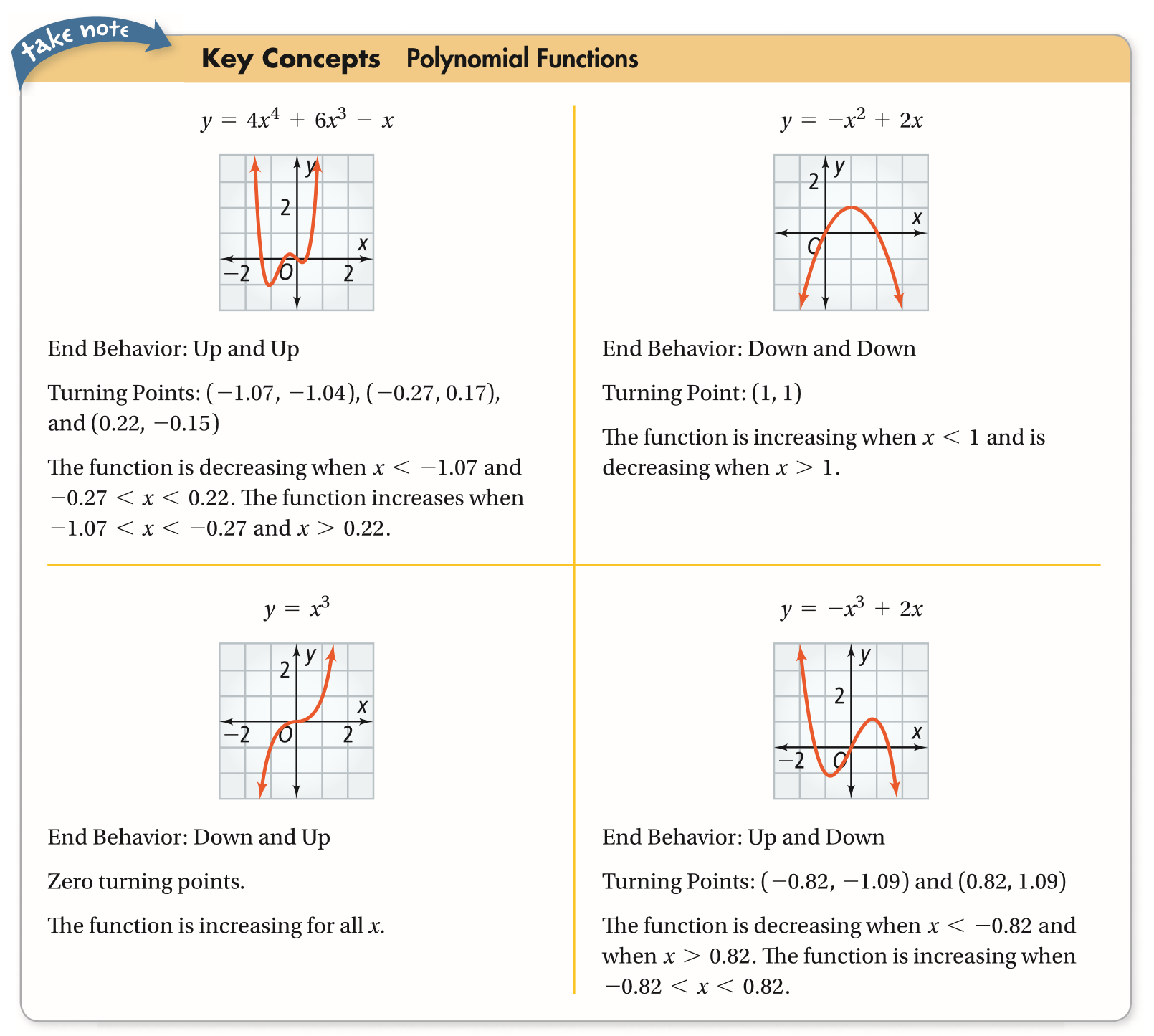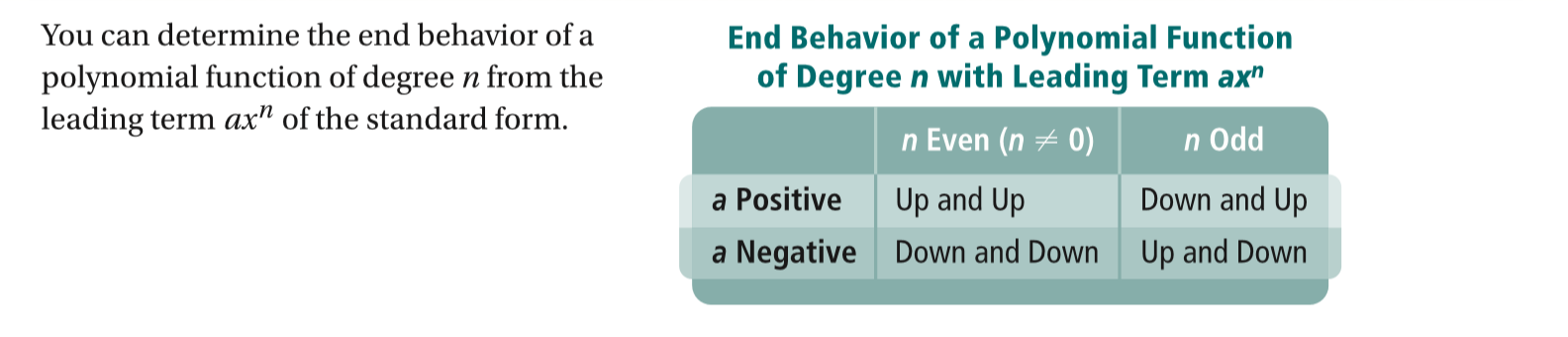28
100 pts
Notes: Take a clear picture or screenshot of your Cornell notes for this lesson. Upload it to the canvas. Zoom and pan as needed.

For a refresher on the Cornell note-taking system, click here.
29
10 pts
Reflection: Math Success
Add to my formatives list

Formative uses cookies to allow us to better understand how the site is used. By continuing to use this site, you consent to the Terms of Service and Privacy Policy.